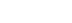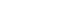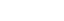xSpecific rotationEncyclopedia
In stereochemistry
Stereochemistry
Stereochemistry, a subdiscipline of chemistry, involves the study of the relative spatial arrangement of atoms within molecules. An important branch of stereochemistry is the study of chiral molecules....

, the specific rotation of a chemical compound
Chemical compound
A chemical compound is a pure chemical substance consisting of two or more different chemical elements that can be separated into simpler substances by chemical reactions. Chemical compounds have a unique and defined chemical structure; they consist of a fixed ratio of atoms that are held together...

[α] is defined as the observed angle of optical rotation
Optical rotation
Optical rotation is the turning of the plane of linearly polarized light about the direction of motion as the light travels through certain materials. It occurs in solutions of chiral molecules such as sucrose , solids with rotated crystal planes such as quartz, and spin-polarized gases of atoms...

α when plane-polarized light is passed through a sample with a path length of 1 decimeter
Decimetre
A decimetre is a unit of length in the metric system, equal to one tenth of a metre, the SI base unit of length. In simple words there are 10 cm in a decimetre....

and a sample concentration of 1 gram
Gram
The gram is a metric system unit of mass....

per 1 millilitre. It is the main property used to quantify the chirality
Chirality (chemistry)
A chiral molecule is a type of molecule that lacks an internal plane of symmetry and thus has a non-superimposable mirror image. The feature that is most often the cause of chirality in molecules is the presence of an asymmetric carbon atom....

of a molecular species or a mineral. The specific rotation of a pure material is an intrinsic property of that material at a given wavelength and temperature. Values should always be accompanied by the temperature at which the measurement was performed and the solvent in which the material was dissolved. Often the temperature is not specified; in these cases it is assumed to be room temperature. The formal unit for specific rotation values is deg dm−1cm3 g−1 but scientific literature uses just degrees
Degree (angle)
A degree , usually denoted by ° , is a measurement of plane angle, representing 1⁄360 of a full rotation; one degree is equivalent to π/180 radians...

. A negative value means levorotatory rotation and a positive value means dextrorotatory rotation. Some examples:
• Sucrose
Sucrose
Sucrose is the organic compound commonly known as table sugar and sometimes called saccharose. A white, odorless, crystalline powder with a sweet taste, it is best known for its role in human nutrition. The molecule is a disaccharide composed of glucose and fructose with the molecular formula...

+66.47°
• Lactose
Lactose
Lactose is a disaccharide sugar that is found most notably in milk and is formed from galactose and glucose. Lactose makes up around 2~8% of milk , although the amount varies among species and individuals. It is extracted from sweet or sour whey. The name comes from or , the Latin word for milk,...

+52.3°
• Cholesterol
Cholesterol
Cholesterol is a complex isoprenoid. Specifically, it is a waxy steroid of fat that is produced in the liver or intestines. It is used to produce hormones and cell membranes and is transported in the blood plasma of all mammals. It is an essential structural component of mammalian cell membranes...

−31.5°
• Camphor
Camphor
Camphor is a waxy, white or transparent solid with a strong, aromatic odor. It is a terpenoid with the chemical formula C10H16O. It is found in wood of the camphor laurel , a large evergreen tree found in Asia and also of Dryobalanops aromatica, a giant of the Bornean forests...

+44.26°
• Penicillin
Penicillin
Penicillin is a group of antibiotics derived from Penicillium fungi. They include penicillin G, procaine penicillin, benzathine penicillin, and penicillin V....

V +223°
• Taxol −49°
• (S)-bromobutane +23.1°
• (R)-bromobutane −23.1°
• (+)-cavicularin
Cavicularin
Cavicularin is a natural phenolic secondary metabolite isolated from the liverwort Cavicularia densa. This macrocycle is unusual because it was the first compound isolated from nature displaying optical activity due to the presence of planar chirality and axial chirality. The specific rotation for...

+168.2°

Optical rotation is measured with an instrument called a polarimeter
Polarimeter
A polarimeter is a scientific instrument used to measure the angle of rotation caused by passing polarized light through an optically active substance....

. There is a linear relationship between the observed rotation and the concentration of optically active compound in the sample. There is a non-linear relationship between the observed rotation and the wavelength of light used.
Specific rotation is calculated using either of two equations, depending on the sample you are measuring:

For pure liquids:In this equation, l is the path length in decimeters, and ρ (Greek letter "rho") is the density of the liquid in g/mL, for a sample at a temperature T (given in degrees Celsius) and wavelength λ (in nanometers). If the wavelength of the light used is 589 nanometer (the sodium D line), the symbol “D” is used. The sign of the rotation (+ or -) is always given.°

For solutions, a different equation is used:In this equation, l is the path length in decimeters and c is the concentration in g/mL, for a sample at a temperature T (given in degrees Celsius) and wavelength λ (in nanometers). If the wavelength of the light used is 589 nanometer (the sodium D line), the symbol “D” is used. The sign of the rotation (+ or –) is always given.
When using this equation, the concentration and the solvent may be provided in parentheses after the rotation. The rotation is reported using degrees, and no units of concentration are given (it is assumed to be g/100mL).

For example:° (c 1.0, EtOH)

This solution equation can also be written as:(concentration in g/mL)

These two forms are identical in practice, and which is more appropriate depends solely on the units of concentration that you are working with.

If a compound has a very large specific rotation or a sample is very concentrated, the actual rotation of the sample may be larger than 180°, and so a single polarimeter measurement cannot detect when this has happened (for example, the values +270° and –90° are not distinguishable, nor are the values 361° and 1°). In these cases, measuring the rotation at several different concentrations allows one to determine the true value. Another method would be to use shorter path-lengths to perform the measurements.

In cases of very small or very large angles, one can also use the variation of specific rotation with wavelength to facilitate measurement. Switching wavelength is particularly useful when the angle is small. Many polarimeters are equipped with a mercury lamp (in addition to the sodium lamp) for this purpose.

The variation of specific rotation with wavelength is the basis of optical rotary dispersion (ORD) that can be used to elucidate the absolute configuration of certain compounds.

Measuring optical rotation provides, in theory, a way to assess optical purity of a sample containing a mixture of enantiomers. For example, if a sample of bromobutane measured under standard conditions has an observed rotation of −9.2°, this indicates that the net effect is due to (100%)(9.2°/23.1°)=40% of the R enantiomer
Enantiomer
In chemistry, an enantiomer is one of two stereoisomers that are mirror images of each other that are non-superposable , much as one's left and right hands are the same except for opposite orientation. It can be clearly understood if you try to place your hands one over the other without...

. The remainder of the sample is a racemic
Racemic
In chemistry, a racemic mixture, or racemate , is one that has equal amounts of left- and right-handed enantiomers of a chiral molecule. The first known racemic mixture was "racemic acid", which Louis Pasteur found to be a mixture of the two enantiomeric isomers of tartaric acid.- Nomenclature :A...

mixture of the enantiomers (30% R and 30% S), which has no net contribution to the observed rotation. The enantiomeric excess
Enantiomeric excess
The enantiomeric excess of a substance is a measure of how pure it is. In this case, the impurity is the undesired enantiomer .-Definition:...

is 40%; the total concentration of R is 70%. However, in practice the utility of this method is limited, as the presence of small amounts of highly rotating impurities can greatly affect the rotation of a given sample. Moreover, the optical rotation of a compound may be non-linearly dependent on its enantiomeric excess because of aggregation in solution. For these reasons other methods of determining the enantiomeric ratio such as gas chromatography or HPLC
High-performance liquid chromatography
High-performance liquid chromatography , HPLC, is a chromatographic technique that can separate a mixture of compounds and is used in biochemistry and analytical chemistry to identify, quantify and purify the individual components of the mixture.HPLC typically utilizes different types of stationary...

with a chiral column is generally preferred.